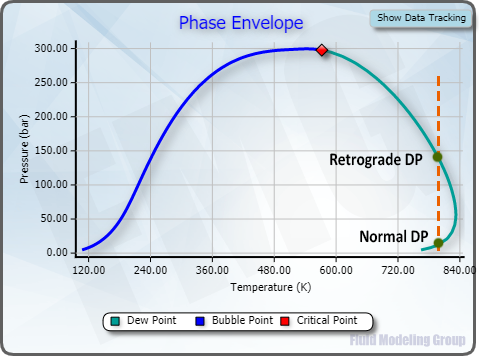### Bubble Point and Dew Point

The Bubble Point is the temperature and pressure at which the first bubble of a vapor appears from a liquid mixture. The Dew Point is the temperature and pressure at which the first “dew” comes out from a gas mixture.

The bubble point temperature may be obtained by heating a liquid at a constant pressure till a first “bubble” come out. It is also the temperature at which the vapor is in equilibrium with the liquid for the given composition. For a pure component, bubble point = boiling point = dew point.

There four (4) types of calculations are provided in this module:

• Bubble Point Temperature: to calculate temperature (T) and vapor compositions (yi) by giving pressure (P) and liquid compositions (xi)
• Bubble Point Pressure: to calculate pressure (P) and vapor compositions (yi) by giving temperature (T) and liquid compositions (xi)
• Dew Point Temperature: to calculate temperature (T) and liquid compositions (xi) by giving pressure (P) and vapor compositions (yi)
• Dew Point Pressure: to calculate pressure (P) and liquid compositions (xi) by giving temperature (T) and vapor compositions (yi)

There are twelve (12) equations of state available for selection combined with the certain mixing rules：• Soave-Redlich-Kwong (SRK), 1972
• Peng-Robinson (PR), 1978
• Peng-Robinson (PR), 1976
• Peng-Robinson (Magoulas & Tassios revision), 1990